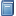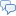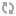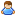BuchempfehlungWindows System Programming
Das Kompendium liefert viele interessante Informationen zur Windows-Programmierung auf Englisch. [Mehr Infos...]FreeBASIC-Chat
Es sindBenutzer im FreeBASIC-Chat online.
(Stand:  )

# fb:porticula NoPaste

## HTTP_Server 

 Uploader:ThePuppetMaster Datum/Zeit: 23.11.2007 18:03:38

```'====================================================================== '(c) 2007 By.: /_\ DeltaLab's - Deutschland 'Autor: Martin Wiemann '====================================================================== Declare Function MkLen(ByRef V_Text as String, ByVal V_Len as Long, ByVal V_AlignRight as uByte) as String Declare Sub TTYDraw() Declare Sub XPrint(V_Text as String) Declare Sub F_Log(ByVal V_Text as String, ByVal V_WhisNow as uByte = 0) Declare Sub F_Header_Create_Thread(V_CID As Any Ptr) #include "vbcompat.bi" #include "dir.bi" #include "tsn.bi" Type MIME_type     V_MIME_Code as String     V_ExecType as String     V_Param as String     V_Exec as Long End Type Type HTTPCode_Side_type     V_HTTPCode as Long     V_SidePath as String End Type Type FIO_type     V_State as Long     V_Path as String     V_FileID as integer     V_AKPos as Long     V_MaxLen as long     V_HeadOnly as Long     V_Rate as Long     V_RateC as Long     V_RateT as Double     V_SData as String End Type Type HTTP_Param_type     V_Name as String     V_Value as String End Type Type HTTP_Type     V_Head as String     V_Data as String     V_DataLen as Long     V_Type as String     V_Request as String     V_RequestSucc as uByte     V_RequestFilename as String     V_RequestParam as String     V_RequestFileType as String     V_RequestMIMEID as Long     V_HTTPVer as String     V_ParamD(25) as HTTP_Param_type     V_ParamC as Long     V_PushPathIn as String     V_PushPathOut as String     V_PushFN as Integer End Type Type Client_Type     V_Thread as Any Ptr     V_Socket as Socket     V_IPA as string     T_Data as String     V_TimeOut as Double     T_HTTP as HTTP_Type     V_FIO as FIO_type End Type Dim X_Server as Socket Dim Shared X_ClientD() as Client_Type Dim Shared T_ClientD as Client_Type Dim Shared X_ClientC as Long Dim Shared G_LogFN as Integer Dim T_Client as Socket Dim BV as Long Dim X as Long Dim Y as Long Dim TIP as string Dim TSock as Socket Dim TThread as any ptr Dim XDrawChangeT as Double Dim TCID as Long Dim TData as String Dim D as String Dim T as String Dim Shared XCC as Long Dim shared XLogDate as String Dim Shared XMIMEPushID as Long Dim Shared XAttrFiles as integer = fbnormal or fbhidden or fbreadonly or fbsystem or fbarchive Dim Shared G_Server_Port as Integer Dim Shared G_Server_MaxClients as Integer Dim Shared G_Server_BlockSize as Long Dim Shared G_Server_TimeOut as Double Dim Shared G_Server_WWWBasePath as String Dim Shared G_Server_LogPath as String Dim Shared G_Server_LogName as String Dim Shared G_MIME_InterpreterD() as MIME_type Dim Shared G_MIME_InterpreterC as Long Dim Shared G_HTTPCode_SidePath as String Dim Shared G_Server_DirList as uByte Dim Shared G_Server_DirListMIME as String Dim Shared G_RootFileRankD() as String Dim Shared G_RootFileRankC as Long #include "HTML_Func.fbmod" #include "HTTP_Func.fbmod" Print "Lade Konfiguration..." G_Server_Port = 1234 '81 G_Server_MaxClients = 10 G_Server_BlockSize = 1024 G_Server_TimeOut = 60 G_Server_WWWBasePath = "" G_HTTPCode_SidePath = "" G_Server_LogPath = "" G_Server_LogName = "" G_MIME_InterpreterC = 0 G_RootFileRankC = 0 Open "Conf.LC1" For Binary as #1 Do     If EOF(1) = -1 then Exit do     Line Input #1, TData     XCC += 1     If TData <> "" then         If Left(TData, 1) <> "'" then             Y = instr(1, TData,"=")             If Y > 0 then                 TIP = Trim(Mid(TData, 1, Y - 1))                 TData = Trim(Mid(TData, Y + 1))                 if TData = "" then TIP = ""                 Select Case lcase(TIP)                     case ""                     case "server_port", "server_maxclients", "server_tx_blocksize", "server_trx_timeout"                         If Val(TData) <> 0 then                             If (CLng(TData) > 0) and (CLng(TData) <= 65535) Then                                 Select Case lcase(TIP)                                     case "server_port": G_Server_Port = CLng(TData)                                     case "server_maxclients": G_Server_MaxClients = CLng(TData)                                     case "server_tx_blocksize": G_Server_BlockSize = CLng(TData)                                     case "server_trx_timeout": G_Server_TimeOut = CLng(TData)                                 End Select                             Else: Print "[LCI] "; lcase(TIP); " Wert muss gröser 0 und kleiner 65536 sein! [Nutze Standardeinstellung]"                             End If                         else: Print "[LCI] "; lcase(TIP); " Wert muss eine Zahl sein! [Nutze Standardeinstellung]"                         Endif                     case "server_path_www", "server_path_httperror"                         If Dir(TData, fbdirectory) <> "" then                             Select Case lcase(TIP)                                 case "server_path_www": G_Server_WWWBasePath = TData                                 case "server_path_httperror": G_HTTPCode_SidePath = TData                             End Select                         else: Print "[LCI] "; lcase(TIP); " Path wurde nicht gefunden / existiert nicht! [Nutze Standardeinstellung]"                         Endif                     case "server_path_log": G_Server_LogPath = exepath & "/" & TData                     case "server_path_log_filename"                         If Len(TData) < 30 then                             for y = 1 to len(TData)                                 Select case asc(mid(TData,y,1))                                     case asc("a") to asc("z")                                     case asc("A") to asc("Z")                                     case asc("0") to asc("9")                                     case asc("_")                                     case asc("-")                                     case else: y = -1: exit for                                 end select                             next                             if y < 0 then                                 Print "[LCI] "; lcase(TIP); " Dateiname enthält ungültige Zeichen [Nutze Standardeinstellung]"                             else: G_Server_LogName = TData                             endif                         else: Print "[LCI] "; lcase(TIP); " Dateiname zu lang! (max. 30 Zeichen) [Nutze Standardeinstellung]"                         endif                     case "server_dir_list": if val(TData) = 1 then G_Server_DirList = 1                     case "server_dir_list_mime": G_Server_DirListMIME = TData                     case "server_root_file"                         G_RootFileRankC += 1                         Redim Preserve G_RootFileRankD(G_RootFileRankC) as String                         G_RootFileRankD(G_RootFileRankC) = TData                     case "mime"                         Y = InStr(1, TData, ",")                         If Y > 0 Then                             D = Trim(Mid(TData, 1, Y - 1))                             TData = Trim(Mid(TData, Y + 1))                             Y = InStr(1, TData, ":")                             If Y > 0 Then                                 T = LCase(Trim(Mid(TData, 1, Y - 1)))                                 TData = Trim(Mid(TData, Y + 1))                                 G_MIME_InterpreterC += 1                                 Redim Preserve G_MIME_InterpreterD(G_MIME_InterpreterC) as MIME_type                                 With G_MIME_InterpreterD(G_MIME_InterpreterC)                                     .V_MIME_Code = lcase(D)                                     .V_ExecType = T                                     .V_Param = TData                                     Select Case T                                         case "mime": .V_Exec = 0                                         case "path": .V_Exec = 1                                     End Select                                 End With                             Else: Print "[LCI] "; lcase(TIP); " Syntaxfehler in MIME Konfiguration!"                             End If                         Else: Print "[LCI] "; lcase(TIP); " Syntaxfehler in MIME Konfiguration!"                         End If                     case else: Print "[LCI] "; lcase(TIP); " Ubekannter Parameter! [Nutze Standardeinstellung]"                 End Select             Else: Print "[LC1] Konfigurationsfehler in Zeile: "; XCC             End if         End if     End If Loop Close #1 X = 0 Y = 0 XCC = 0 if G_RootFileRankC = 0 then     G_RootFileRankC += 1     Redim Preserve G_RootFileRankD(G_RootFileRankC) as String     G_RootFileRankD(G_RootFileRankC) = "index.html" End If F_Log String(50, "=") F_Log "Öffne Log...", 1 XPrint "" If G_Server_WWWBasePath = "" then XPrint "[FATALER FEHLER!] Es wurde kein User-Verzeichniss angegeben! Ohne Verzeichniss kann der Server keine Informationen zur verfügung stellen!" XPrint "" XPrint "Versuche Server zu inizialisieren..." Do     sleep 1000     If InKey = Chr(27) then end     BV = TSN_Create_Listen(X_Server, G_Server_Port)     If BV >= 0 then         XPrint "[OK] HTTP_Server arbeitet, und wartet auf Verbindungsanfragen!"         exit do     End If Loop sleep 1000 Dim THID as Any Ptr Do     If X_Server = 0 Then         BV = TSN_Create_Listen(X_Server, G_Server_Port)         If BV >= 0 then             XPrint "[OK] HTTP_Server arbeitet, und wartet auf Verbindungsanfragen!"             exit do         End If     End If     BV = TSN_Event_Get(X_Server)     Select Case BV         Case 0         Case 1             TCID = 0             For X = 1 To X_ClientC                 If X_ClientD(X).V_Socket <= 0 Then                     TCID = X                     Exit For                 End If             Next             If TCID = 0 Then                 If X_ClientC < G_Server_MaxClients then                     X_ClientC += 1                     TCID = X_ClientC                     Redim Preserve X_ClientD(X_ClientC) as Client_Type                 End If             End If             BV = TSN_Create_Accept(X_Server, T_Client)             If BV = 0 then                 If TCID > 0 then                     X_ClientD(TCID) = T_ClientD                     X_ClientD(TCID).V_Socket = T_Client                     BV = TSN_IPAddress_Get(T_Client, TIP)                     If BV = 0 then X_ClientD(TCID).V_IPA = TIP                     X_ClientD(TCID).V_TimeOut = Timer + G_Server_TimeOut                     THID = ThreadCreate(@F_Header_Create_Thread, @TCID)                     Sleep 1                     If THID = 0 Then TSN_Close T_Client                     X_ClientD(X).V_Thread = THID                     Sleep 1                 Else                     XPrint "[REQ] No Free -> Create New -> SERVER FULL! KILL NEW!"                     TSN_Data_Send T_Client, HTTP_Header_Create(307, HTML_HTTPCode_CreatePage(307, HTTP_Get_Code_Desc(307)))                     TSN_Close T_Client                 End If             Else                 XPrint "[Fehler] " & TSN_GuruCode("DE", BV)                 Exit Do             End If         Case Else             XPrint "[Fehler] " & TSN_GuruCode("DE", BV)             Exit Do     End Select     If XDrawChangeT < Timer then         TTYDraw         XDrawChangeT = Timer + 0.20     end if Loop Until InKey = Chr(27) TSN_Close X_Server For X = 1 to X_ClientC     TSock = X_ClientD(X).V_Socket     TSN_Close TSock     ThreadWait(X_ClientD(X).V_Thread) Next sleep 1 if BV <> 0 then Print "[Fehler] "; TSN_GuruCode("DE", BV) F_Log "Schliesse Log...", 1 F_Log String(50, "=") if G_LogFN <> 0 then close #G_LogFN End BV Sub TTYDraw() Dim XX as Long Dim XW as Long Dim XH as Long Dim XS as Long Dim XV as Long DIM TS as String Dim DD() as String DIM TW as String XW = LOWORD(Width) XH = HIWORD(Width) Redim DD(X_ClientC) as String Dim TClient as Client_Type For XX = 1 to X_ClientC     TClient = X_ClientD(XX)     With TClient         TW = Format(.V_TimeOut - Timer, "000") & "-" & TClient.V_Socket         If .V_Socket > 0 then             Select Case TClient.V_FIO.V_State                 case 0: TS = "[" & MKLen(str(XX), 5, 1) & "][" & MKLen(.V_IPA, 15, 1) & "][    ][             ][" & TW & "][" & String(25, 32) & "] Warte auf eingehende Daten..."                 case 1: TS = "[" & MKLen(str(XX), 5, 1) & "][" & MKLen(.V_IPA, 15, 1) & "][    ][             ][" & TW & "][" & String(25, 32) & "] Lese Anfrage / Erzeuge Antwort..."                 case 2: TS = "[" & MKLen(str(XX), 5, 1) & "][" & MKLen(.V_IPA, 15, 1) & "][    ][             ][" & TW & "][" & String(25, 32) & "] Sende HTTP-Header..."                 case 3                     XV = Fix(100 / .V_FIO.V_MaxLen * .V_FIO.V_AKPos)                     TS = "[" & MKLen(str(XX), 5, 1) & "][" & MKLen(.V_IPA, 15, 1) & "][" & MKLen(str(XV) & "%", 4, 1) & "][" & MKLen(Format(.V_FIO.V_Rate, "###,###,###"), 8, 1) & " KB/s][" & TW & "][" & MKLen(String(Fix(XV / 4), 35), 25, 0) & "] " & .T_HTTP.V_RequestFilename                 case 9: TS = "[" & MKLen(str(XX), 5, 1) & "][" & MKLen(.V_IPA, 15, 1) & "][    ][             ][" & TW & "][" & String(25, 32) & "] Schliesse Verbindung..."                 case else: TS = "[" & MKLen(str(XX), 5, 1) & "][" & MKLen(.V_IPA, 15, 1) & "][    ][             ][" & TW & "][" & String(25, 32) & "] =[UNBEKANNTER STATUS]="             End Select '           If (X_ClientD(XX).V_TimeOut - Timer) < 0 Then '               TSN_Close TClient.V_Socket '               X_ClientD(XX) = TClient '           End If         else: TS = "[" & MKLen(str(XX), 5, 1) & "][" & String(15, 32) & "][    ][             ][   ][" & String(25, 32) & "] -"         End if         If Len(TS) > XW then TS = Left(TS, XW)         DD(XX) = TS & String(XW - len(TS), 32)     End With Next XS = XH - X_ClientC - 2 For XX = XCC to X_ClientC     Print "" Next XCC = X_ClientC + 2 Locate XS, 1, 0 For XX = 1 to X_ClientC     Print DD(XX) Next Print Format(Now, "yyyy.mm.dd - hh:mm:ss") end sub Sub XPrint(V_Text as String) Dim XW as Long Dim XH as Long Dim XS as Long XW = LOWORD(Width) XH = HIWORD(Width) XS = XH - X_ClientC - 3 Locate XH, 1, 0 Print "" Locate XS, 1, 0 Print V_Text & String(XW - Len(V_text), 32) F_Log V_Text End Sub Function MkLen(ByRef V_Text as String, ByVal V_Len as Long, ByVal V_AlignRight as uByte) as String If Len(V_Text) < V_len then     If V_AlignRight = 1 then         Return String(V_Len - Len(V_Text), 32) & V_Text     else: Return V_Text & String(V_Len - Len(V_Text), 32)     Endif else: Return V_Text endif end Function Sub F_Log(ByVal V_Text as String, ByVal V_WhisNow as uByte = 0) If XLogDate <> format(now, "yyyy_mm_dd") then     XLogDate = format(now, "yyyy_mm_dd")     if G_LogFN <> 0 then Close #G_LogFN: G_LogFN = 0     if G_Server_LogPath <> "" then         If dir(G_Server_LogPath, fbdirectory) = "" then             XPrint "LOG-Path existiert nicht! Erstelle Path! -> " & G_Server_LogPath             MKDir G_Server_LogPath             If dir(G_Server_LogPath, fbdirectory) = "" then XPrint "[=FEHLER=] Konnte Log-Verzeichniss nicht erstellen!"         end if         G_LogFN = FreeFile         Open G_Server_LogPath & "/" & G_Server_LogName & XLogDate & ".log" for binary as #G_LogFN     Endif End If if G_LogFN <> 0 then     Dim T as String     T = V_Text & chr(13) & chr(10)     If V_WhisNow = 1 then T = "[" & format(now, "yyyymmdd-hhmmss-") & str(timer) & "] " & T     Put #G_LogFN, Lof(G_LogFN) + 1, T End if end sub Sub F_Header_Create_Thread(V_CID As Any Ptr) Dim X As Integer = *cptr(Integer Ptr, V_CID) Dim TSock as Socket Dim TBV as Long Dim TCID as Long Dim TData as String X_ClientD(X).V_TimeOut = Timer + G_Server_TimeOut * 10 do         TSock = X_ClientD(x).V_Socket         If TSock > 0 Then             If (X_ClientD(x).V_TimeOut - Timer) >= 0 then                 Select case X_ClientD(x).V_FIO.V_State                     case 0                         TBV = TSN_Data_Get(Tsock, TData)                         Select Case TBV                             Case 0                             case 2                                 X_ClientD(X).V_TimeOut = Timer + G_Server_TimeOut                                 X_ClientD(x).T_Data += TData                                 HTTP_Header_Read x                             Case 3: X_ClientD(x).V_FIO.V_State = 9                             case else                                 XPrint "[Fehler] " & TSN_GuruCode("DE", TBV)                                 X_ClientD(x).V_FIO.V_State = 9                         End Select                 End Select                 Select case X_ClientD(x).V_FIO.V_State                     case 1                         Dim T as String                         Dim XFT as String                         XFT = X_ClientD(X).T_HTTP.V_RequestFileType                         X_ClientD(X).V_FIO.V_State = 2                         If Right(X_ClientD(X).V_FIO.V_Path,1) = "/" then                             XPrint "[" & format(now, "yyyymmdd-hh:mmss") & "][" & MKLen(X_ClientD(X).V_IPA, 15, 1) & "][" & MKLen(X_ClientD(X).T_HTTP.V_Type,4,1) & "][" & str(200) &"] " & X_ClientD(X).T_HTTP.V_Request                             XMIMEPushID += 1                             T = ExePath & "/MIME_PUSH/"                             If Dir(T, fbdirectory) = "" then MKDir T                             X_ClientD(X).T_HTTP.V_PushPathOut = T & str(XMIMEPushID) & ".MIMEOUT"                             X_ClientD(X).V_FIO.V_FileID = Freefile                             Open X_ClientD(X).T_HTTP.V_PushPathOut for Binary as #X_ClientD(X).V_FIO.V_FileID                             Put #X_ClientD(X).V_FIO.V_FileID, 1, HTTP_Header_Create(200, HTML_HTTP_CreateDirList(G_Server_WWWBasePath, X_ClientD(X).V_FIO.V_Path, X_ClientD(X).T_HTTP.V_Request), , "text/html")                             Close #X_ClientD(X).V_FIO.V_FileID                             X_ClientD(X).V_FIO.V_Path = X_ClientD(X).T_HTTP.V_PushPathOut                             X_ClientD(X).V_FIO.V_FileID = Freefile                             Open X_ClientD(X).V_FIO.V_Path for Binary as #X_ClientD(X).V_FIO.V_FileID                             X_ClientD(X).V_FIO.V_MaxLen = LOF(X_ClientD(X).V_FIO.V_FileID)                             If X_ClientD(X).V_FIO.V_HeadOnly = 1 then X_ClientD(X).V_FIO.V_State = 9                         else                             X_ClientD(X).V_FIO.V_FileID = Freefile                             Open X_ClientD(X).V_FIO.V_Path for Binary as #X_ClientD(X).V_FIO.V_FileID                             X_ClientD(X).V_FIO.V_MaxLen = LOF(X_ClientD(X).V_FIO.V_FileID)                             If X_ClientD(X).V_FIO.V_HeadOnly = 1 then X_ClientD(X).V_FIO.V_State = 9                             TSock = X_ClientD(X).V_Socket                             TSN_Data_Send TSock, HTTP_Header_Create(200, "", X_ClientD(X).V_FIO.V_MaxLen, MIME_Get_Type_Desc(XFT))                         end if                         XPrint "[" & format(now, "yyyymmdd-hh:mmss") & "][" & MKLen(X_ClientD(X).V_IPA, 15, 1) & "][" & MKLen(X_ClientD(X).T_HTTP.V_Type,4,1) & "][" & str(200) &"] " & X_ClientD(X).T_HTTP.V_Request                         X_ClientD(X).V_FIO.V_AKPos = 1                         X_ClientD(X).V_FIO.V_State = 3                     case 2                     case 3                         X_ClientD(X).V_TimeOut = Timer + G_Server_TimeOut                         if X_ClientD(x).V_Socket <> 0 then                             TData = Space(G_Server_BlockSize)                             If X_ClientD(x).V_FIO.V_MaxLen - X_ClientD(x).V_FIO.V_AKPos < G_Server_BlockSize then TData = Space(X_ClientD(x).V_FIO.V_MaxLen - X_ClientD(x).V_FIO.V_AKPos + 1)                             Get #X_ClientD(x).V_FIO.V_FileID, X_ClientD(x).V_FIO.V_AKPos, TData                             TSN_Data_Send TSock, TData                             X_ClientD(x).V_FIO.V_AKPos = X_ClientD(x).V_FIO.V_AKPos + Len(TData)                             X_ClientD(x).V_FIO.V_RateC = X_ClientD(x).V_FIO.V_RateC + Len(TData)                             If X_ClientD(x).V_FIO.V_RateT < Timer then                                 X_ClientD(x).V_FIO.V_Rate = (X_ClientD(x).V_FIO.V_RateC * 2) \ 2000                                 X_ClientD(x).V_FIO.V_RateC = 0                                 X_ClientD(x).V_FIO.V_RateT = Timer + 0.5                             End If                             If X_ClientD(x).V_FIO.V_MaxLen <= X_ClientD(x).V_FIO.V_AKPos then X_ClientD(x).V_FIO.V_State = 9                             X_ClientD(TCID).V_TimeOut = Timer + G_Server_TimeOut                         else: X_ClientD(x).V_FIO.V_State = 9                         End if                     case 9                         if X_ClientD(x).V_FIO.V_FileID <> 0 then Close #X_ClientD(x).V_FIO.V_FileID                         if X_ClientD(X).T_HTTP.V_PushPathIn <> "" then                             Kill X_ClientD(X).T_HTTP.V_PushPathIn                             X_ClientD(X).T_HTTP.V_PushPathIn = ""                         End if                         if X_ClientD(X).T_HTTP.V_PushPathOut <> "" then                             Kill X_ClientD(X).T_HTTP.V_PushPathOut                             X_ClientD(X).T_HTTP.V_PushPathOut = ""                         End if                         X_ClientD(x).V_FIO.V_FileID = 0                         TSN_Close TSock                         X_ClientD(x).V_Socket = TSock                 end select             Else                 XPrint "TimeOut!" '               If X_ClientD(x).V_FIO.V_State <> 1 then                     TSN_Close TSock                     X_ClientD(x).V_Socket = TSock '               Else: HTTP_SendErrorClose X, 408 '               End if             End if         Else: Exit Do         End If loop TSN_Close TSock TSock = 0 X_ClientD(X).V_Socket = TSock end Sub```